# 【JavaSE】数组的定义和使用

1、数组的基本概念

1.1、什么是数组

1.2、数组的创建及初始化

1.2.1、数组的创建

1.2.2、数组的初始化

1.3、数组的使用

1.3.1、数组中元素的访问

1.3.2、遍历数组

2、数组是引用类型

2.1、初始JVM的内存分布

2.2、基本类型变量与引用类型变量的区别

2.3、再谈引用变量

2.4、认识null

3、数组的应用场景

3.1、保存数据

3.2、作为函数的参数

3.2.1、参数传基本数据类型

3.2.2、参数传数组类型（引用数据类型）

3.3、作为函数的返回值

4、数组练习

4.1、数组转字符串

4.2、数组拷贝

4.2.1、调用copeOf:

# 1、数组的基本概念

## 1.1、什么是数组

1. 数组中存放的元素其类型相同
2. 数组的空间是连在一起的
3. 每个空间有自己的编号，起始位置的编号为0，即数组的下标。

## 1.2、数组的创建及初始化

### 1.2.1、数组的创建

T [ ] 数组名 = new T [N];

T: 表示数组中存放元素的类型

T [ ] :表示数组的类型

N : 表示数组的长度

``````int[] array1 = new int;       //创建一个可以容纳10个int类型元素的数组
double[] array2 = new double;  //创建一个可以容纳5个double类型的数组
String[] array3 = new String;  //创建一个可以容纳3个字符串的数组``````

其中 int[ ]、double[ ]、String[ ]均表示数组的类型

### 1.2.2、数组的初始化

1、动态初始化：在创建数组时，直接指定数组中的元素个数

``````int[]array = new int;
``````

2、静态初始化：在创建数组时不直接指定数据的元素个数，而直接将具体的数据内容进行指定

T[ ] 数组名称 = {data1,data2,......datan};

``````int[]array1 = new int[]{1,2,3,4,5,6,7,8,9};
double[]array2 = new double[]{1,2,3,4,5,6};
String[] array3 = new String[]{"hello","java","!!!"};``````

• 静态初始化虽然没有指定数组的长度，编译器在编译时会根据{}中元素个数来确定数组的长度。
• 静态初始化时, {}中数据类型必须与[]前数据类型一致
• 静态初始化可以简写，省去后面的new T[]
``````// 注意：虽然省去了new T[], 但是编译器编译代码时还是会还原
int[] array1 = {0,1,2,3,4,5,6,7,8,9};
double[] array2 = {1.0, 2.0, 3.0, 4.0, 5.0};
String[] array3 = {"hell", "Java", "!!!"};``````
• 静态和动态初始化可以分为两步但是省略格式不可以
``````int[] array1;
array1 = new int;//动态

int[] array2;
array2 = new int[]{10, 20, 30};//静态

// 注意省略格式不可以拆分, 否则编译失败
// int[] array3;
// array3 = {1, 2, 3}//这样是不行的``````
• 如果没有对数组进行初始化，数组中元素有其默认值

1、 如果数组中存储元素类型为基本类型，默认值为基本类型对应的默认值，比如：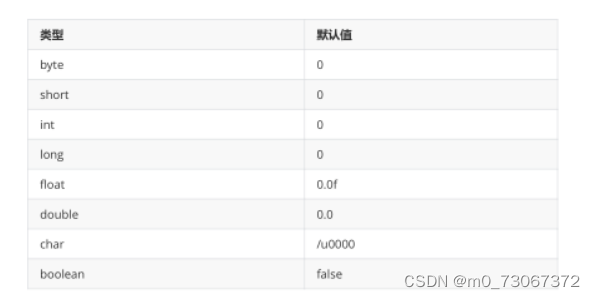2、如果数组中存储元素类型为引用类型，默认值为null

``````    public static void main(String[] args) {
boolean[] array = new boolean;//false
char[] array2 = new char;//'\u0000'

String str = new String("hello");
String str2 = "hello";//这两句代码效果一样

System.out.println("========");
}``````

## 1.3、数组的使用

### 1.3.1、数组中元素的访问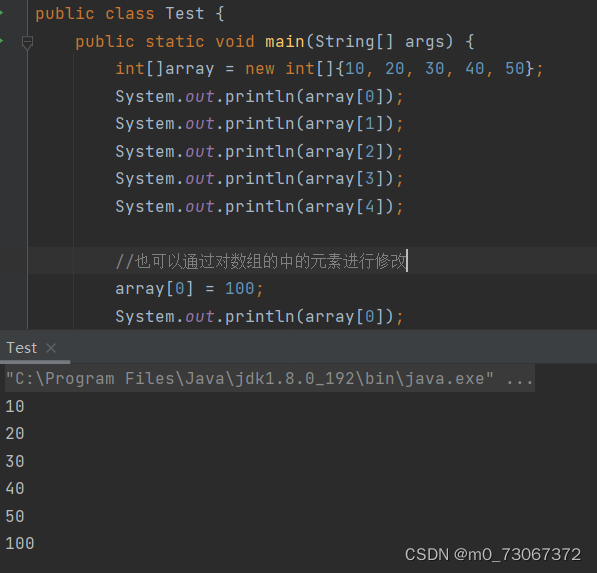【注意事项】：

1. 数组是一段连续的内存空间，因此支持随机访问，即通过下标访问快速访问数组中任意位      置的元素
2. 下标从0开始，介于[0, N）之间不包含N，N为元素个数，不能越界，否则会报出下标越界       异常。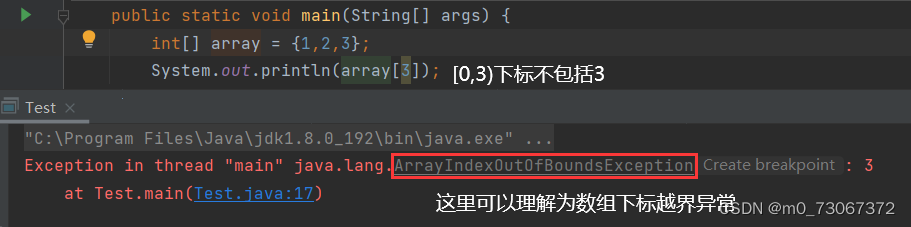### 1.3.2、遍历数组

``````int[]array = new int[]{10, 20, 30, 40, 50};
System.out.println(array);
System.out.println(array);
System.out.println(array);
System.out.println(array);
System.out.println(array);``````

1. 如果数组中增加了一个元素，就需要增加一条打印语句
2. 如果输入中有100个元素，就需要写100个打印语句
3. 如果现在要把打印修改为给数组中每个元素加1，修改起来非常麻烦。

``````int[]array = new int[]{10, 20, 30, 40, 50};
for(int i = 0; i < 5; i++){
System.out.println(array[i]);
}``````

改成循环之后，上述三个缺陷中问题2和3可以解决，但是无法解决问题1。那能否获取到数组的长度呢？

``````int[]array = new int[]{10, 20, 30, 40, 50};
for(int i = 0; i < array.length; i++){
System.out.println(array[i]);
``````

`````` public static void main(String[] args) {
int[] array = {1,2,3};
for(int x:array){
System.out.println(x);
}
}
``````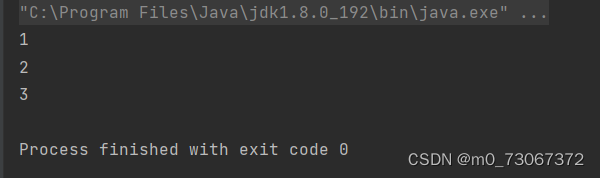for-each 是 for 循环的另一种使用方式，能够更方便的完成对数组的遍历，可以避免循环条件和更新语句写错

# 2、数组是引用类型

## 2.1、初始JVM的内存分布

内存是一段连续的存储空间，主要用来存储程序运行时数据。

1. 程序运行时代码需要加载到内存
2. 程序运行产生的中间数据要存放在内存
3. 程序中的常量也要保存
4. 有些数据可能需要长时间存储，而有些数据当方法运行结束后就要被销毁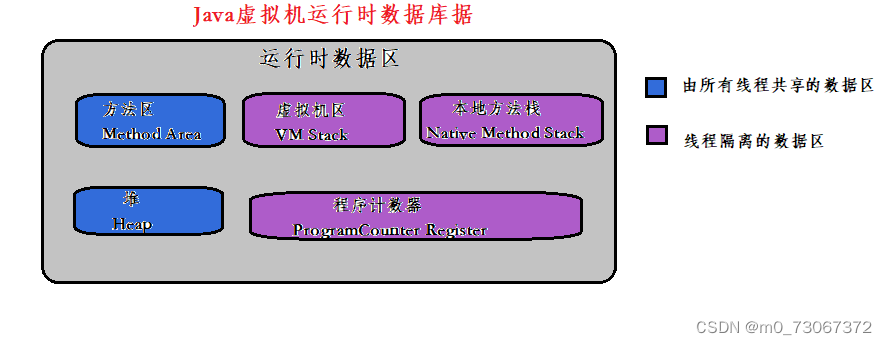•  程序计数器 (PC Register): 只是一个很小的空间, 保存下一条执行的指令的地址
• 虚拟机栈(JVM Stack): 与方法调用相关的一些信息，每个方法在执行时，都会先创建一个栈帧，栈帧中包含有：局部变量表、操作数栈、动态链接、返回地址以及其他的一些信息，保存的都是与方法执行时相关的一些信息。比如：局部变量。当方法运行结束后，栈帧就被销毁了，即栈帧中保存的数据也被销毁了。
• 本地方法栈(Native Method Stack): 本地方法栈与虚拟机栈的作用类似. 只不过保存的内容是Native方法的局部变量. 在有些版本的 JVM 实现中(例如HotSpot), 本地方法栈和虚拟机栈是一起的
• (Heap): JVM所管理的最大内存区域. 使用 new 创建的对象都是在堆上保存 (例如前面的 new int[]{1, 2,3} )，堆是随着程序开始运行时而创建，随着程序的退出而销毁，堆中的数据只要还有在使用，就不会被销毁。
• 方法区(Method Area): 用于存储已被虚拟机加载的类信息、常量、静态变量、即时编译器编译后的代码等数据. 方法编译出的的字节码就是保存在这个区域

## 2.2、基本类型变量与引用类型变量的区别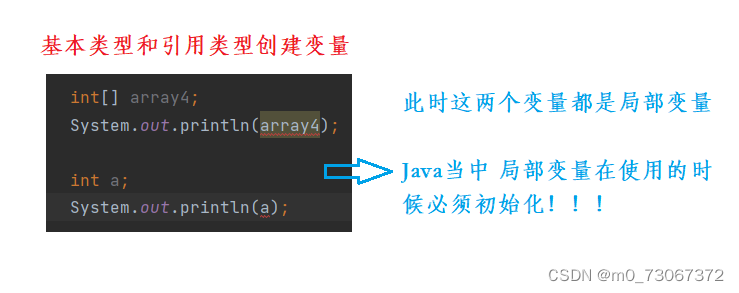``````public static void func() {
int a = 10;
int b = 20;
int[] arr = new int[]{1,2,3};
}``````

a、b内置类型的变量，因此其空间中保存的就是给该变量初始化的值
array数组类型的引用变量，其内部保存的内容可以简单理解成是数组在堆空间中的首地址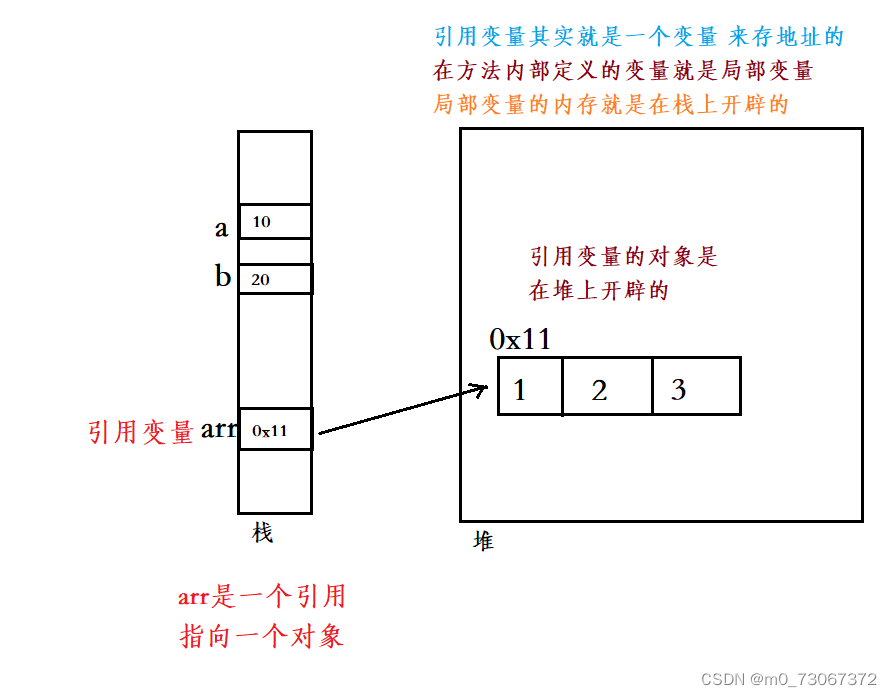总结：引用变量引用类型创建的一个变量不能和局部变量相提并论；在一个方法中创建引用变量，那么这个引用变量就是局部变量

从上图可以看到引用变量不直接存储对象本身，可以简单理解成存储的是对象在堆中空间的起始地址。通过该地址，引用变量便可以去操作对象。有点类似C语言中的指针，但是Java中引用要比指针的操作更简单。

## 2.3、再谈引用变量

``````import java.util.Arrays ;
public class Test {
public static void main(String[] args) {
int[] array1 = {1,2,3,4};
int[] array2 = {11,22,33,44};
array1 = array2 ;
array1  = 1888;
System.out.println(Arrays.toString(array1));
System.out.println(Arrays.toString(array2));

}
}``````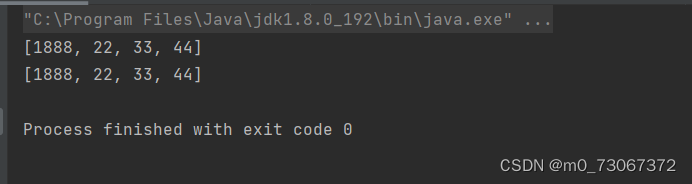为什么是这个结果呢？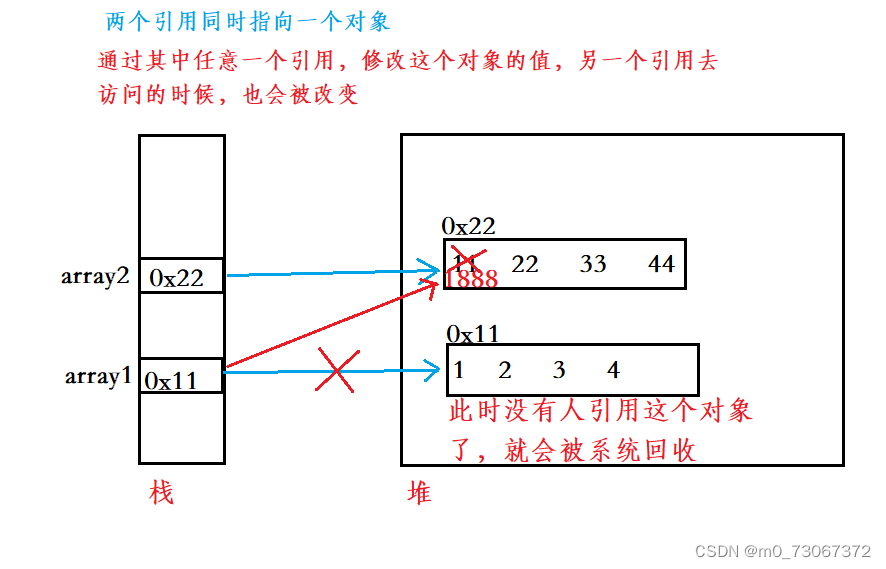## 2.4、认识null

null 在 Java 中表示 "空引用" , 也就是一个不指向对象的引用

``````int[]arr = null;//arr这个引用不指向任何一个对象，所以在写的时候用null赋值
System.out.println(arr);``````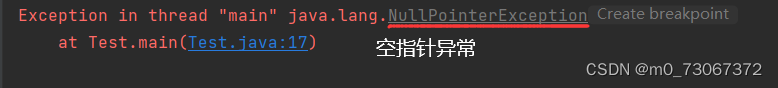null 的作用类似于 C 语言中的 NULL (空指针), 都是表示一个无效的内存位置. 因此不能对这个内存进行任何读写操作. 一旦尝试读写, 就会抛出 NullPointerException————>空指针异常。

注意: Java 中并没有约定 null 和 0 号地址的内存有任何关联

# 3、数组的应用场景

## 3.1、保存数据

``````    public static void main(String[] args) {
int[] array = {1, 2, 3};
for(int i = 0; i < array.length; ++i){
System.out.println(array[i] + " ");
}
}``````

## 3.2、作为函数的参数

### 3.2.1、参数传基本数据类型

``````public static void main(String[] args) {
int num = 0;
func(num);//这里num为实参
System.out.println("num = " + num);
}

public static void func(int x) {//这里x为形参
x = 10;
System.out.println("x = " + x);
}

// 执行结果
x = 10
num = 0``````

### 3.2.2、参数传数组类型（引用数据类型）

``````    public static void func1(int[] array) {//array为形参
array = new int[]{15,16,17};
//从这里下面 都改变了实参的值了
}

public static void func2(int[] array) {//array为形参
array = 999;
}
public static void main(String[] args) {
int[] array1 = {1,2,3,4};
func1(array1);
//func2(array1);//实参
System.out.println(Arrays.toString(array1));
}``````

结果是：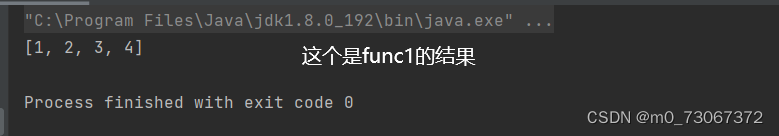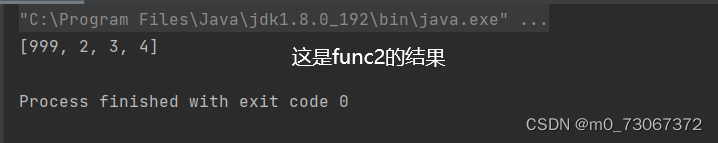为什么呢？

func1中改变了形参的指向，但是没有改变实参的指向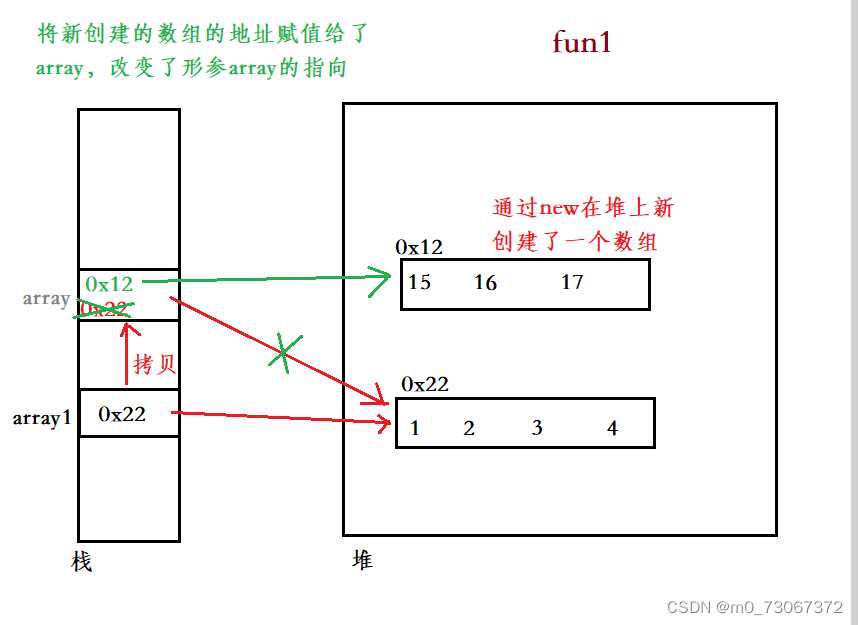func2中array1只是将引用（数组地址）传给array，通过引用array改变数组的值，两个引用同时指向同一个对象（数组）。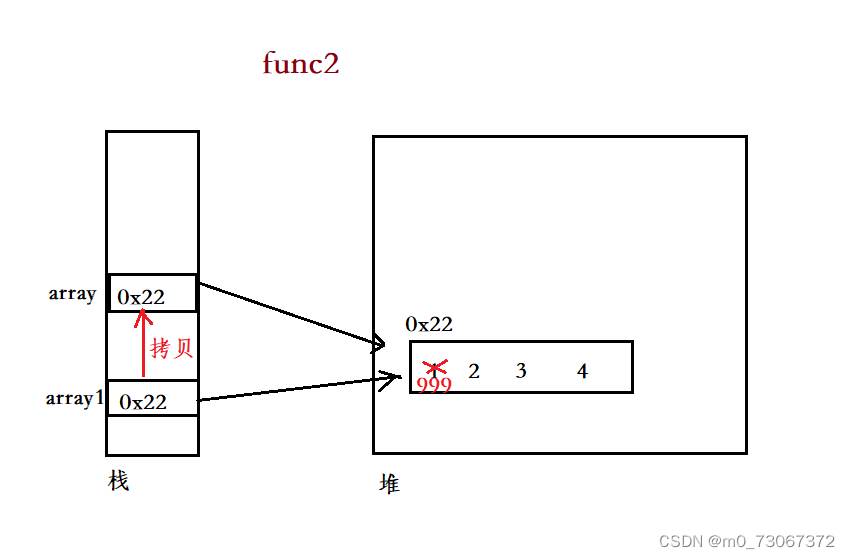1. 形参修改指向：array = new int;    通过在形参array所在范围内，new一个新对象，                           将原引用覆盖，达到修改形参指向的目的。只会影响形参的指向
2. 形参修改指向对象的值：array = 9;  此时才会影响到实参

## 3.3、作为函数的返回值

``````import java.util.Arrays;
public class Test2 {
public static void main(String[] args) {
int[] array = func1();
System.out.println(Arrays.toString(array));
}
public static int[] func1(){
int[] tmpArr = {10,11,12};
return tmpArr;//将数组当成返回值返回
}``````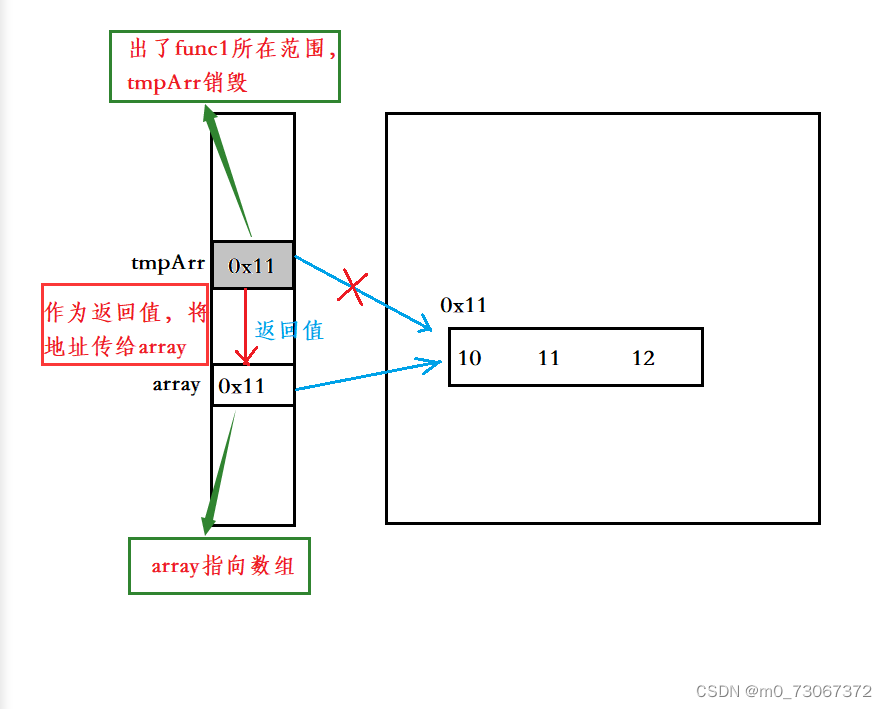再看一个代码：引用（数组）作为参数和返回值

``````import java.util.Arrays;
public class Test2 {
public static void main(String[] args) {
int[] array = {1,2,3,4,5,6,7,8,9};
int[] ret = grow(array);
System.out.println(Arrays.toString(array));
System.out.println(Arrays.toString(ret));
}
public static int[] grow(int[] array1){
for(int i = 0; i < array1.length; i++){
array1[i] = array1[i]*2;
}
return array;
}``````

这里为什么两个输出结果是一样的？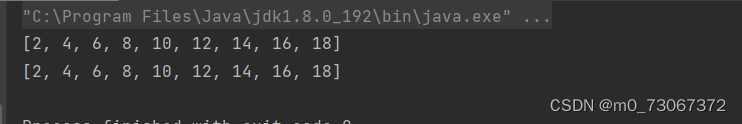画图解释：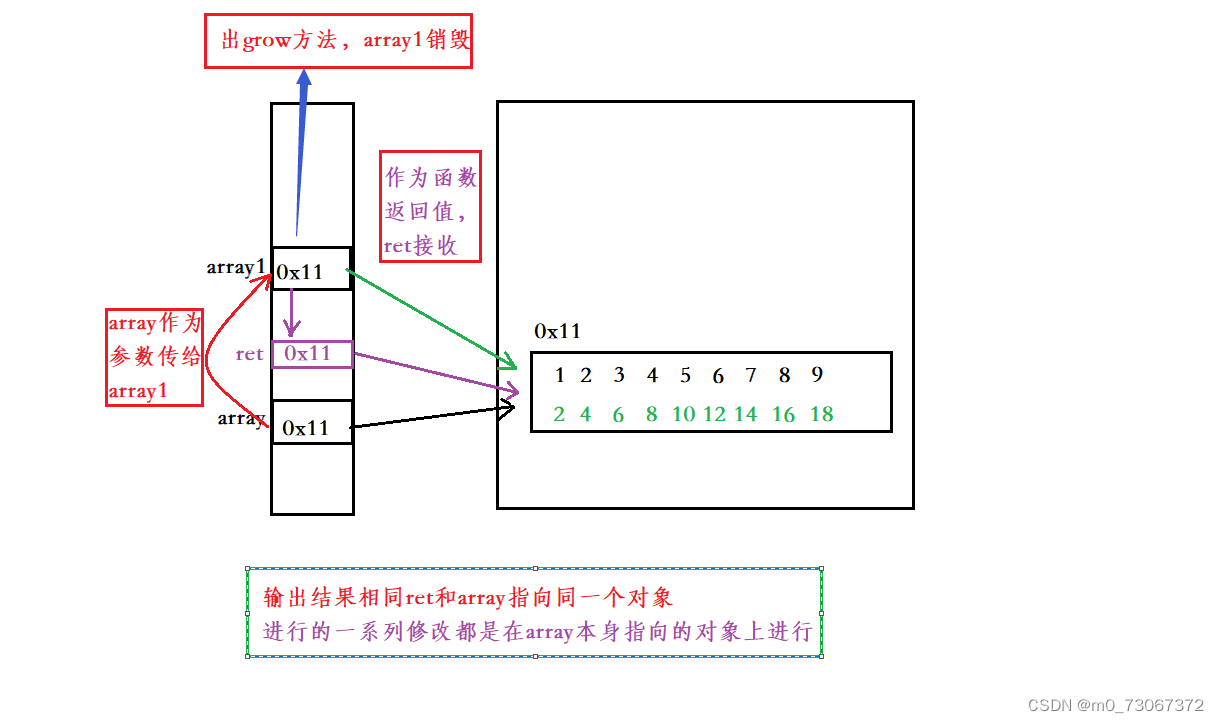既然ret和array指向同一个对象，那么就可以将个代码进行优化：

``````import java.util.Arrays;
public class Test2 {
public static void main(String[] args) {
int[] array = {1,2,3,4,5,6,7,8,9};
grow2(array);//这里不设置变量来接收返回值，
System.out.println(Arrays.toString(array));

}
public static void grow(int[] array1) {//这里也可以设置成void类型的返回值，不进行返
//回
for (int i = 0; i < array1.length; i++) {
array1[i] = array1[i] * 2;
}
}
//这样修改的原因是：grow方法在原对象上修改，没设置新对象。``````

``````import java.util.Arrays;
public class Test2 {
public static void main(String[] args) {
int[] array ={1,2,3,4,5,6,7,8};
int[] ret = grow3(array);
System.out.println(Arrays.toString(array));
System.out.println(Arrays.toString(ret));
}
public static int[] grow3(int[] array1){
int[] tmpArray = new int[array1.length];
for (int i = 0; i < array1.length; i++) {
tmpArray[i] = array1[i]*2;
}
return tmpArray;
}
``````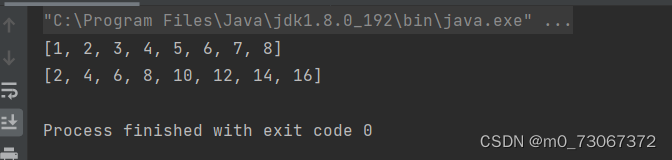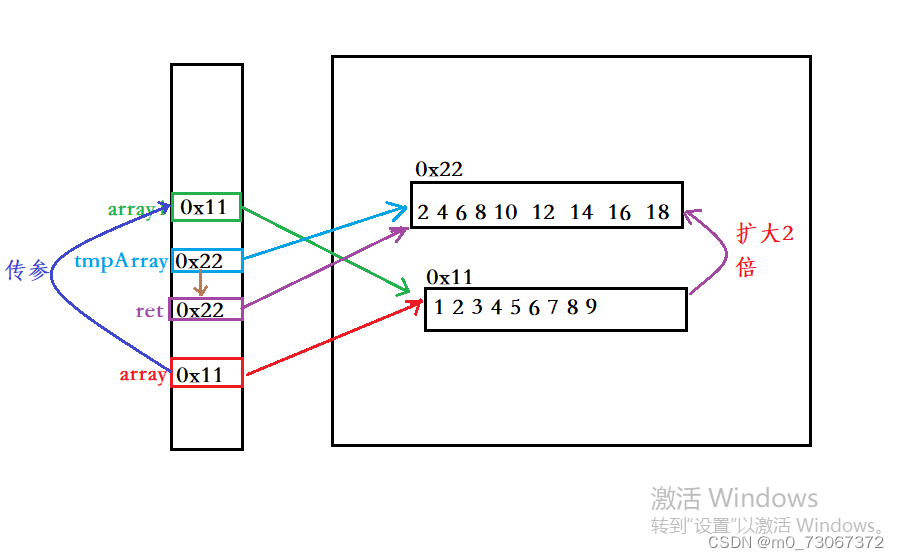# 4、数组练习

## 4.1、数组转字符串

``````import java.util.Arrays;
public class Test2 {
public static void main(String[] args) {
int[] array = {1,2,3,4,5};
System.out.println(Arrays.toString(array));//通过toString将array以字符串的形式
//输出
}``````

``````public class Test2 {
public static String myToString(int[] array){
if(array == null){
return "null";//返回字符串null
}
String ret = "[";//在开始前加[
for (int i = 0; i < array.length ; i++) {
// ret += array[i]+",";//数字遍历完一个数字之后拼接一个,
ret += array[i];
if(i !=array.length -1){
ret +=" , ";
}
}
ret = ret +"]";//这里是在输出完之后拼接了一个]
return ret;
}
public static void main(String[] args) {
int[] array = {1,2,3,4,5};
System.out.println(myToString(array) );//通过toString将array以字符串的形式输出
System.out.println(myToString(null) );
}

//结果为：[1,2,3,4,5]
//        null``````

返回值类型设为String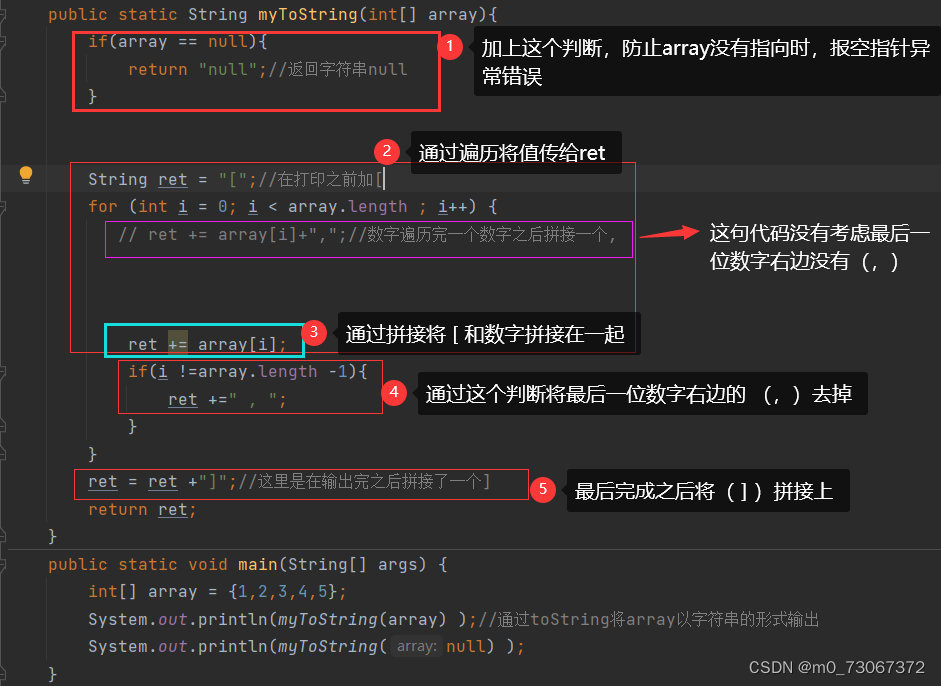写出上述代码的历程：

1、思考将数组以字符串的形式打印出来，[1,2,3,4,5 ]

2、那么[ ]就应该和数字拼接在一起

3、数字就应该是通过遍历得来的，

4、每个数字在遍历完之后应该在后边拼接一个（，）

5、考虑到5右边没有（，），那么就应该通过if语句5右边的（，）去掉

6、最后在使用时，考虑到引用没有指向时，编译器会报空指针异常，所以添加标记1

## 4.2、数组拷贝

``````import java.util.Arrays;
public class Test2 {
public static void main(String[] args) {
int[] array = {1,2,3,4};
int[] array2 = new int[array.length];
for (int i = 0; i < array.length; i++) {
array2 [i] = array[i];
}
System.out.println(Arrays.toString(array));
System.out.println(Arrays.toString(array2));
System.out.println("============");
array2  =199;
System.out.println(Arrays.toString(array));
System.out.println(Arrays.toString(array2));
}``````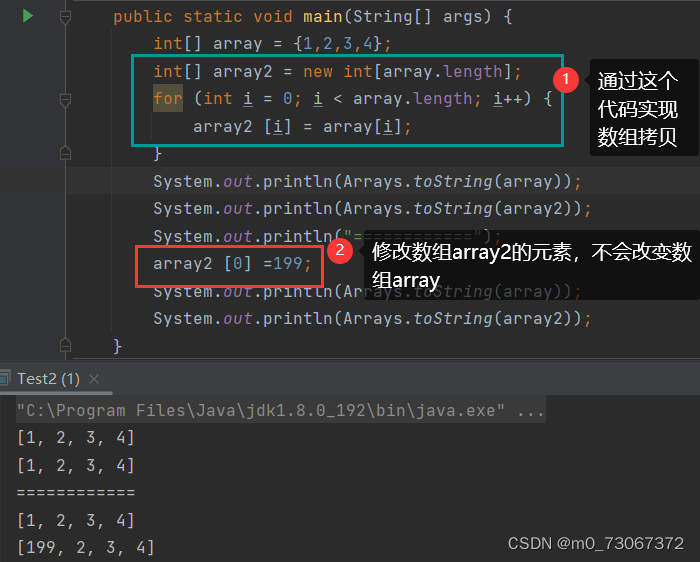上边的拷贝方式太过复杂，在Java当中JDK提供了拷贝的方法

### 4.2.1、调用copeOf: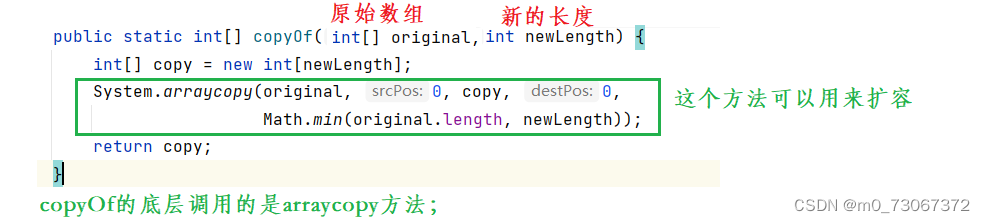1、 使用copyOf来实现拷贝

``````import java.util.Arrays;
public class Test2 {
public static void main(String[] args) {
int[] array = {1,2,3,4};
int[] ret = Arrays.copyOf(array,array.length);
System.out.println(Arrays.toString(array));
System.out.println(Arrays.toString(ret));
System.out.println("============");
ret  =199;
System.out.println(Arrays.toString(array));
System.out.println(Arrays.toString(ret));
}``````

2、使用arraycopy来进行拷贝

``````import java.util.Arrays;
public class Test2 {
public static void main(String[] args) {
int[] array = {1,2,3,4};
int[] copy = new int[array.length];
System.arraycopy(array,1,copy,0,array.length-1) ;
System.out.println(Arrays.toString(array));
System.out.println(Arrays.toString(copy));
System.out.println("============");
copy  =199;
System.out.println(Arrays.toString(array));
System.out.println(Arrays.toString(copy));
}``````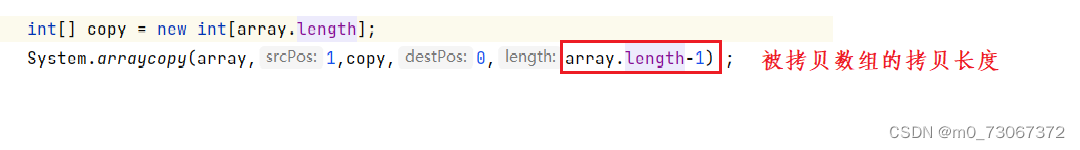总结：

1、通过for循环来拷贝

2、通过copyOf来拷贝

3、通过arraycopy来拷贝，他的优点是可以指定区间进行拷贝！！

当然拷贝的方式还有很多。

3、 也可以通过copyOf来进行扩容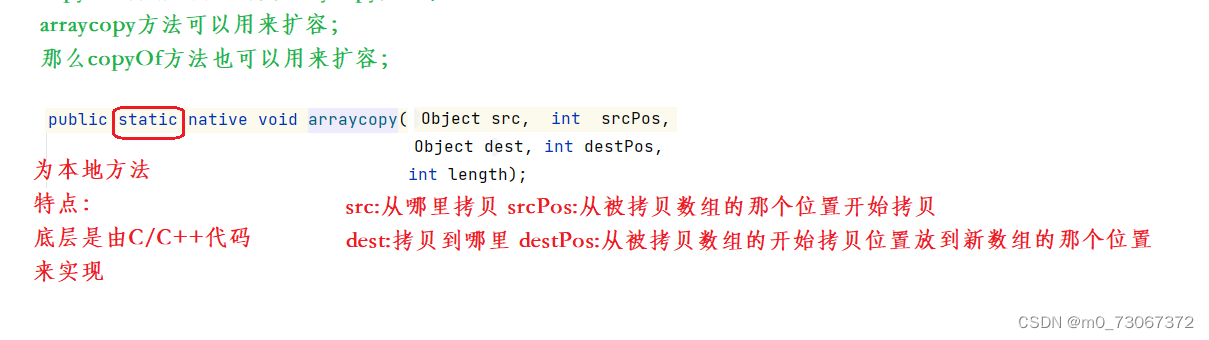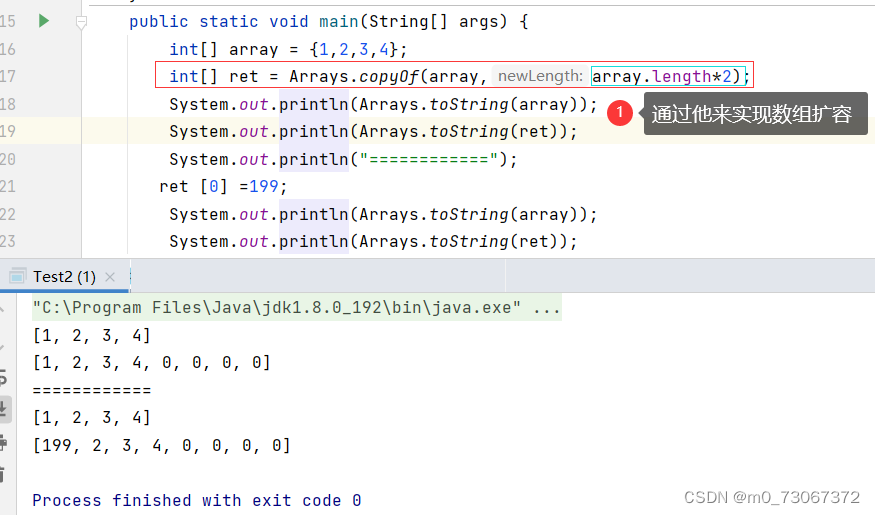4、 调用copyOfRang————》将指定数组的指定范围复制到新数组中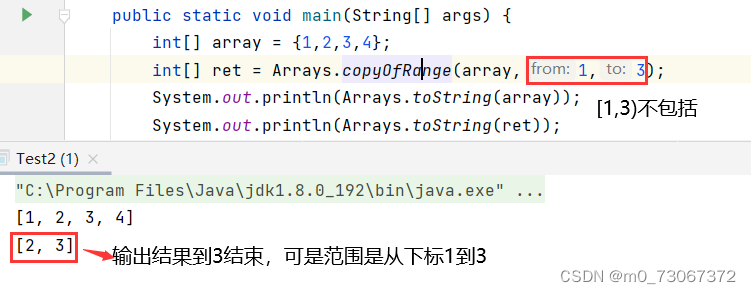注意：大多数方法中（from      to    ）表示的是左闭右开。